# CREST Reasoning Olympiad Class 3 Sample Papers

## Syllabus:

Section 1: Patterns, Analogy and Classification, Geometrical Shapes, Mirror Images and Water Images, Direction Sense Test, Ranking Test, Alphabet Test and Logical Sequence of Words, Puzzle Test, Coding - Decoding.

Achievers Section: Higher Order Thinking Questions - Syllabus as per Section 1

 Q.1 What number completes the Puzzle?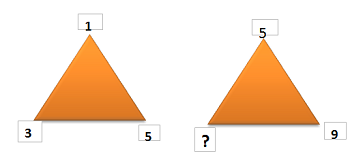Q.2 What numbers will you add along the sides of each of the triangle in order to get the number in the center?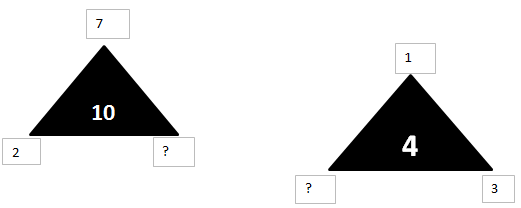Q.3 If cushion is called pillow, pillow is called mat, mat is called bed sheet, and bed sheet is called cover, which will be used under the head while sleeping?
 Q.4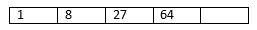Q.5 Arrange the following in a logical order of drawing in a drawing class: 1. Colour. 2. Use Sketch Pen. 3. Draw. 4. Show to Teacher.
 Q.6 Ravi left home and cycled 10 km towards South, then turned right and cycled 5 km and then again turned right and cycled 10 km. After this he turned left and cycled 10 km. How many kilometers will he have to cycle to reach his home straight?
 Q.7 What number completes the Puzzle ?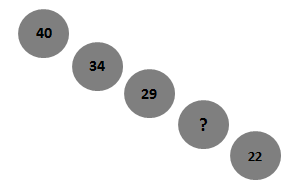Q.8 Each of the following questions is based on the following information: 1. A # B means B is at 1 meter to the right of A. 2. A \$ B means B is at 1 meter to the North of A. 3. A * B means B is at 1 meter to the left of A. 4. A @ B means B is at 1 meter to the south of A. 5. In each question first person from the left is facing North. According to X @ B * P, P is in which direction with respect to X?
 Q.9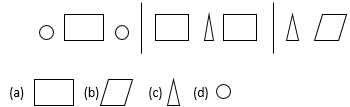Q.10 ZA: YB: ? : WD

Sample PDF of CREST Reasoning Olympiad for Class 3: